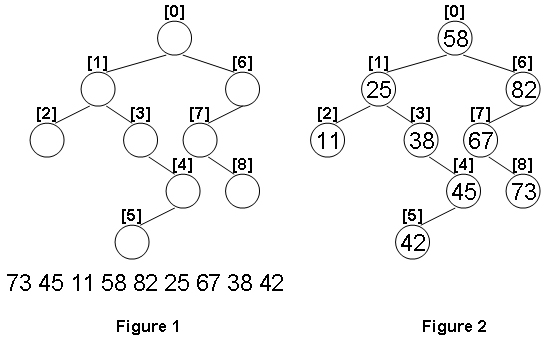A Binary Search Tree (BST) is recursively defined as a binary tree which has the following properties:

The left subtree of a node contains only nodes with keys less than the node's key.
The right subtree of a node contains only nodes with keys greater than or equal to the node's key.
Both the left and right subtrees must also be binary search trees.
Given the structure of a binary tree and a sequence of distinct integer keys, there is only one way to fill these keys into the tree so that the resulting tree satisfies the definition of a BST. You are supposed to output the level order traversal sequence of that tree. The sample is illustrated by Figure 1 and 2.## Input Specification:

Each input file contains one test case. For each case, the first line gives a positive integer N (≤100) which is the total number of nodes in the tree. The next N lines each contains the left and the right children of a node in the format left_index right_index, provided that the nodes are numbered from 0 to N−1, and 0 is always the root. If one child is missing, then −1 will represent the NULL child pointer. Finally N distinct integer keys are given in the last line.

## Output Specification:

For each test case, print in one line the level order traversal sequence of that tree. All the numbers must be separated by a space, with no extra space at the end of the line.

## Sample Input:

9
1 6
2 3
-1 -1
-1 4
5 -1
-1 -1
7 -1
-1 8
-1 -1
73 45 11 58 82 25 67 38 42

## Sample Output:

58 25 82 11 38 67 45 73 42

## 解答

#include<vector>
#include<iostream>
#include<algorithm>

using namespace std;

struct node { int key, l, r, level; };

vector<node> nodes;
vector<int> keys;
int cnt = 0;

void createTree(int root, int level) {
if (root != -1) {
createTree(nodes[root].l, level + 1);
nodes[root] = { keys[cnt++], nodes[root].l, nodes[root].r, level };
createTree(nodes[root].r, level + 1);
}
}

int main()
{
int n;
scanf("%d", &n);
nodes.resize(n);
keys.resize(n);
for (int i = 0; i < n; i++)
scanf("%d %d", &nodes[i].l, &nodes[i].r);
for (int i = 0; i < n; i++)
scanf("%d", &keys[i]);
sort(keys.begin(), keys.end());
createTree(0, 0);
sort(nodes.begin(), nodes.end(), [](node a, node b) {
return a.level == b.level ? a.key < b.key : a.level < b.level;
});
printf("%d", nodes.begin()->key);
for (int i = 1; i < nodes.size(); i++)
printf(" %d", nodes[i].key);
return 0;
}